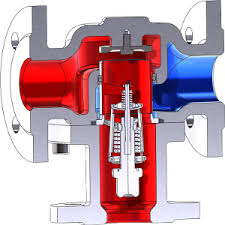# Control valve sizing – Equations & its importance# What is valve sizing & it’s importance?

The can be defined as picking the right size valve for the job or, in certain instances where a valve size is predestined, the correct trim size.

Valve sizing procedures are based on accepted mathematical equations that are used to model flow through ideal restrictions such as orifice plates and flow nozzles. The sizing equations are based on equations for predicting the flow of compressible and incompressible fluids through control valves.

### Undersizing:

Undersizing leads to the limited amount of fluid supply. Limited capacity may result in process failure because of the inability to supply needed fluids in sufficient quantity. Undersized can also result in safety hazards; for example, an undersized control valve that is used in a relief application may allow upstream pressure to reach unsafe levels.

### Oversizing:

A valve with excess capacity may spend most of its life throttling near the seat. Sustained throttling with the plug near the seat causes high-velocity flow that impinges on and around the seating surfaces. The oversizing cause high process gains and it will cause instability of the process.

## Valve flow coefficient Cv:

The valve flow coefficient, CV, is the number of U.S. gallons per minute of water at 60°F that will pass through a given flow restriction with a pressure drop of one psi. Cv that combined the flow area of the valve orifice, the contraction coefficient, and the head loss coefficient all in one:Where:
q = The volumetric flow rate.
N1 = A numerical constant for units of measurement (see Figure 4).
Cv = The control valve flow coefficient.
Gf = The liquid specific gravity at upstream conditions; the ratio of the fluid density at the valve inlet to the density of water at 60 degrees F (15.6 degrees C).
p1 = The upstream absolute pressure, psia.
p2 = The downstream absolute pressure, psia

But for critical flow condition, the valve which seems to block the flow passages. What really happens is that at a certain ratio of pressure drop to inlet pressure defined by a pressure recovery factor FL^2 the flow becomes choked at the orifice. (FL originally was called the “critical flow factor,”2 Cf and was used as such.)### For Liquid Service:

Volumetric Flow:
Is if ΔP is less than FL^2 (ΔPs)for critical flow:

Occurs when ΔP is more than FL^2 (ΔPs)Flow by Weightfor critical flow:

Occurs when ΔP is more than FL^2 (ΔPs)Gf = specific gravity @ flowing temperature (water = 1 @ 60°F)
P1 = absolute inlet pressure, psia
P2 = absolute outlet pressure, psia
ΔP = P1 – P2
Pc = pressure at thermodynamic critical point,
Pv = vapor pressure of liquid at flowing temperature,
q = liquid flow rate, U.S. gpm
W = flow in pounds per hour

Scroll to Top